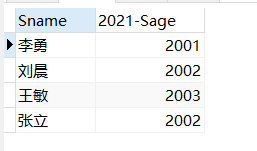# 数据库系统（一）——数据查询

#### 一、实验目的：

1. 熟练掌握SQL定义数据表和索引的方法；

2. 能够使用SQL完成数据的单表查询、多表查询和嵌套查询操作。

#### 二、实验内容：

1. 根据指定场景创建数据库；

2. 根据具体的查询应用需求写出相应的SQL查询语句，并得到正确的查询结果。
1、熟悉基于单表的SQL的数据查询功能；
2、掌握基于单表的GROUP BY子句、HAVING子句、ORDER BY子句的用法；
3、掌握基于单表的（NOT）IN等谓词的用法，掌握集合函数的用法；
4、掌握多表连接查询的适用情况和语句构造方法；

#### 三、实验过程：

##### 1、创建学生课程数据库 （stu_course）：
``create database stu_course; ``
##### 2、学生表、课程表和学生选课表的创建和修改：
###### 2.1、创建数据表：
``````create table Student(
Sno char(9)primary key,
Sname char(20)unique,
Ssex char(2),
Sage smallint,
Sdept char(20)
);

create table Course (
Cno char(4)primary key,
Cname char(4) not null,
Cpno char(4),
Ccredit smallint ,
foreign key(Cpno) references Course(Cno)
);

create table SC(
Sno char(9),
Cno char(4),
primary key(Sno,Cno),
foreign key(Sno) references Student(Sno),
foreign key(Cno) references Course(Cno)
);``````
###### 2.2、修改数据表：

``alter table Student add S_entrance Date;``

``alter table Student alter column Sage int;``

``alter table Course add unique(Cname);``

``drop  table Student;``
##### 3、插入数据：
``````INSERT INTO student VALUES ('201215121', '李勇', '男', 20, 'CS');
INSERT INTO student VALUES ('201215122', '刘晨', '女', 19, 'CS');
INSERT INTO student VALUES ('201215123', '王敏', '女', 18, 'MA');
INSERT INTO student VALUES ('201215125', '张立', '男', 19, 'IS');

INSERT INTO course VALUES ('1', '数据库', '5', 4);
INSERT INTO course VALUES ('2', '数学', NULL, 2);
INSERT INTO course VALUES ('3', '信息系统', '1', 4);
INSERT INTO course VALUES ('4', '操作系统', '6', 3);
INSERT INTO course VALUES ('5', '数据结构', '7', 4);
INSERT INTO course VALUES ('6', '数据处理', NULL, 2);
INSERT INTO course VALUES ('7', 'PASCAL语言', '6', 4);

INSERT INTO sc VALUES ('201215121', '1', 92);
INSERT INTO sc VALUES ('201215121', '2', 85);
INSERT INTO sc VALUES ('201215121', '3', 88);
INSERT INTO sc VALUES ('201215122', '2', 90);
INSERT INTO sc VALUES ('201215122', '3', 80);``````
##### 4、各种类型的查询操作：
###### 4.1 、单表查询操作：

1、查询指定列

``select Sno,Sname from Student;``

2、查询全部列

``select * from Student;``

3、查询经过计算的值

``select Sname 2021-Sage from Student;``4、消除出重复的元组

``select Distinct Sno from Student; ``

5、查询满足条件的元组

``select Sname from Student where sdept='CS';``

``select * from student where Sage <20;``

``select sname,sdept,sage from student where sage between 20 and 23; ``

``select sname,sdept,sage from student where sage not between 20 and 23; ``

6、确定集合（IN）

``select Sname,Ssex from student where Sdept in('CS','MA','IS'); ``

``select Sname,Ssex from student where Sdept not in('CS','MA','IS'); ``

7、字符匹配（模糊查询）

``````like ：字符串匹配
% :代表任意长度字符
_ :代表单个字符``````

``select * from where Sno like '201215121';``

``select Sname,Sno,Ssex from student where Sname like'刘%'``

``select Sname,Sno,Ssex from student where Sname not like'刘%'``

``select Sname,Sno from student where Sname like'_阳%';``

8、多重条件查询（and ，or）

``select Sname from student where Sdept='CS' and Sage < '20';``

9、order by

``select Sno,Grade from SC where Cno='3' order by Grade DESC;``

10、聚集函数

``````count(*)                     统计元组个数
count([distinct|all] <列名>)  统计一列中值的个数
Sum([distinct|all] <列名>)    求一列总和
Max([distinct|all] <列名>)    求一列中的最大值
Min([distinct|all] <列名>)    求一列中的最小值
Avg([distinct|all] <列名>)    求一列的平均值``````

``select count(*) from student;``

``select count(distinct Sno) from student;``

``select sum(Ccredit) from SC,Course where Sno='201215121' and SC.Cno = Course.Cno;``

11、分组（group by）

``select Cno,count(*) from SC group by Cno;``

``select Sno from SC group by Sno having count(*)>1;``

###### 4.2 、多表连接查询操作：

``select student.*,SC.* from student,SC where student.Sno=SC.Sno; ``

``select student.Sno,Sname from Student,SC where Student.Sno=SC.Sno and SC.Cno='2' and SC.Grade>90;``

#### 四、总结：

• HAVING 是跟GROUP BY 连在一起用的，放在GROUP BY 后面，此时的作用相当于WHERE。

• WHERE 后面的条件中不能有聚集函数，比如SUM(),AVG()等，而HAVING 可以

×